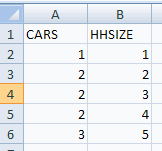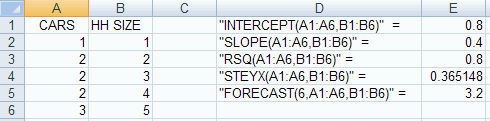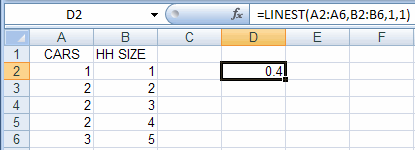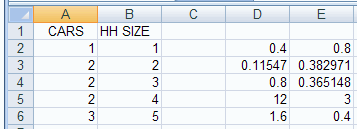## A. Colin Cameron, Dept. of Economics, Univ. of Calif. - Davis

This January 2009 help sheet gives information on
• Fitting a regression line using Excel functions INTERCEPT, SLOPE, RSQ, STEYX and FORECAST.
• Fitting a regression line using Excel function LINEST.
• Prediction using Excel function TREND.
For most purposes these Excel functions are unnecessary.
It is easier to instead use the Data Analysis Add-in for Regression.

REGRESSION USING EXCEL FUNCTIONS INTERCEPT, SLOPE, RSQ, STEYX and FORECAST

The data used are in carsdata.xlsThe population regression model is:    y = β1 + β2 x + u

We wish to estimate the regression line:     y = b1 + b2 x

The individual functions INTERCEPT, SLOPE, RSQ, STEYX and FORECAST can be used to get key results for two-variable regression

• INTERCEPT(A1:A6,B1:B6) yields the OLS intercept estimate of 0.8
• SLOPE(A1:A6,B1:B6) yields the OLS slope estimate of 0.4
• RSQ(A1:A6,B1:B6) yields the R-squared of 0.8
• STEYX(A1:A6,B1:B6) yields the standard error of the regression of 0.36515  0.8
• FORECAST(6,A1:A6,B1:B6) yields the OLS forecast value of Yhat=3.2 for X=6 (forecast 3.2 cars for household of size 6).Thus the estimated model is
y = 0.8 + 0.4*x
with R-squared of 0.8 and estimated standard deviation of u of 0.36515
and we forecast that for  x = 6 we have y = 0.8 + 0.4*6 = 3.2.

REGRESSION USING EXCEL FUNCTION LINEST

The individual function LINEST can be used to get regression output similar to that
several forecasts from a two-variable regression.

This is tricky to use.
The formula leads to output in an array (with five rows and two columns (as here there are two regressors), so we need to use an array formula.

We consider an example where output is placed in the array D2:E6.

First in cell D2 enter the function LINEST(A2:A6,B2:B6,1,1).Then
• Highlight the desired array D2:E6
• Hit the F2 key  (Then edit appears at the bottom left of the dpreadsheet).Finally Hit CTRL-SHIFT-ENTER.
This yieldswhere the results in A2:E6 represent
Slope coeff        Intercept coeff
St.error of slope  St.error of intercept
R-squared          St.error of regression
F-test overall     Degrees of freedom (n-k)
Regression SS      Residual SS

In particular, the fitted regression is
CARS = 0.4 + 0.8 HH SIZE   with R2 = 0.8
The estimated coefficients have standard errors of, respectively, 0.11547 and 0.382971.

To get just the coefficients give the LINEST command with the last entry 0 rather than 1, ie. LINEST(A2:A6,B2:B6,1,0),
and then highlight cells A8:B8, say, hit F2 key, and hit CTRL-SHIFT-ENTER.LINEST can be extended to multiple regression (more than an intercept and one regressor).
Then the first two rows of output are extended to number of columns equals number of regressors (including constant)
and the last three rows are the same as earlier.

The LOGEST function is the same as the LINEST function, except that an exponential relationship is estimated rather than a linear relationship.

PREDICTION USING EXCEL FUNCTION TREND

The individual function TREND can be used to get several forecasts from a two-variable regression.
This is tricky to use:

• Set up the X values for the forecast, say 6 in cell C2 and 7 in cell C3.
• In cell A8 give the function TREND(A2:A6,B2:B6,C2:C3,1).
• Note that labels are not included when using function TREND.
This gives only one value of 3.2 in cell B21. We need the other forecast as well.• Highlight cells A8:A9 and hit the F2 key (then Edit appears at the bottom of the screen).
• Hit CTRL-SHIFT-ENTER.Thus for X=6 we forecast Y=3.2, and for X=7 we forecast Y=3.6, as expected given Y = 0.8 + 0.4*X.

Function TREND can be extended to multiple regression (more than an intercept and one regressor).

For further information on how to use Excel go to
http://cameron.econ.ucdavis.edu/excel/excel.html